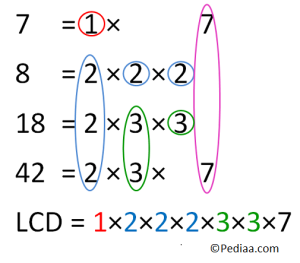# How to Find the Least Common Denominator

Denominator is the lower part of a vulgar fraction. i.e. a fraction given in the form a/b , where b is the denominator. A common denominator is a common multiple of all the denominators of two or more vulgar fractions. Specifically, the lowest common denominator or least common denominator (LCD) is the important one. Least common multiple of all the denominators is known as the lowest common denominator. To find common denominator or to find the least common denominator there are several methods.

## Calculate the Least Common Denominator

Method 1.

Consider the fractions 1/2 and 1/3. The denominators are 2 and 3. To find the common denominators, we need multiples of 2 and 3.
List the multiples of 2 and 3 in two separate rows.

2→ 2,4,6,8,10,12,14,16…..
3→3,6,9,12,15,18,21…..

We can see 6 and 12 are included in both rows. Therefore, they are multiples of both 2 and 3. However, the smaller of the two is 6, and it is called the least common multiple of 2 and 3. 12 is also a multiple but not the lowest. Therefore, 6 is the LCD of 2 and 3. Then we can write 1/2 and 1/3 as equivalent fractions with 6 in the denominator. This allows addition and subtraction to be performed on the two fractions easily.

1/2 = 3/6 and 1/3 = 2/6

Then 1/2 +1/3 = 3/6 + 2/6 = 5/6  and  1/2 – 1/3 = 3/6 – 2/6 = 1/6

Method 2.

Above method is inefficient when larger numbers are involved. Therefore, we have to use prime factoring to obtain the common denominators.

Consider the fractions 1/7, 1/8, 1/18 and 1/42. (Obviously determining the multiples of each denominator and selecting the common one is going to be difficult than the earlier one)

First write the denominators as a product of their prime factors. (Any real number can be written as a product of prime numbers). Then we have,

7 = 1×7
8 = 2×2×2
18 = 2×3×3
42 = 2×3×7

Select the primes present in the numbers. For above examples, 1,2,3, and 7 are the primes in the above numbers. Multiply these primes up to the greatest number occurring in each denominator (for example 2 is used thrice in 8; therefore, the multiple should 2 three times. Similarly, 3 is used twice in 18; therefore, the product should include 3 two times)

The least common multiple of 7,8,18 and 42 is

= 1×2×2×2×3×3×7 = 504Therefore, the Lowest Common Denominator is 504 and 1/7, 1/8, 1/18 and 1/42 can be given as equivalent fractions 72/504, 63/504, 28/504, 12/504